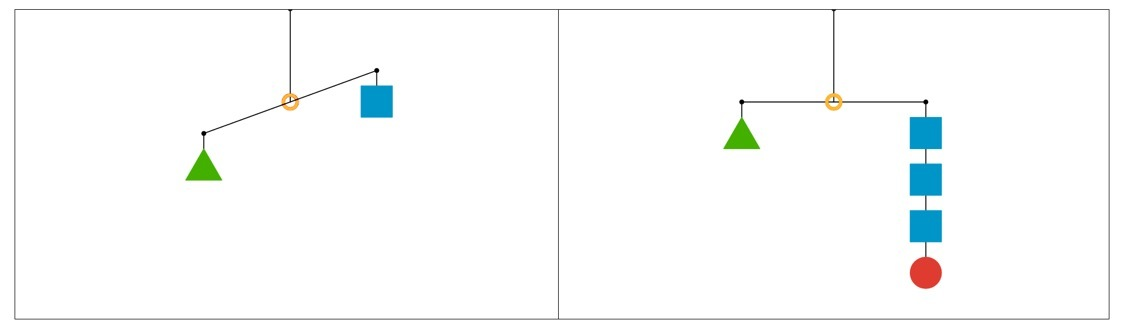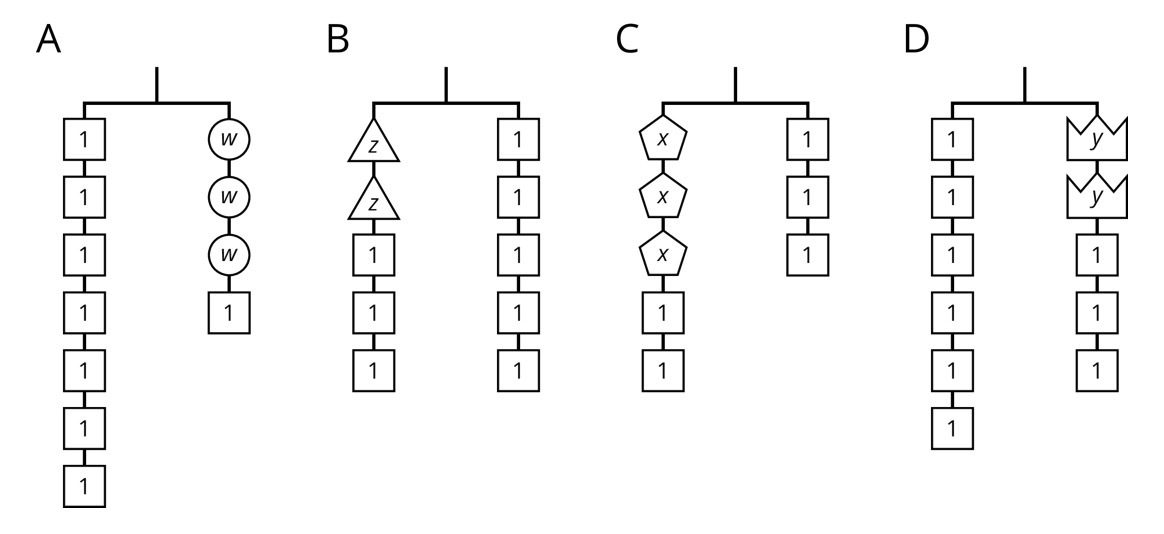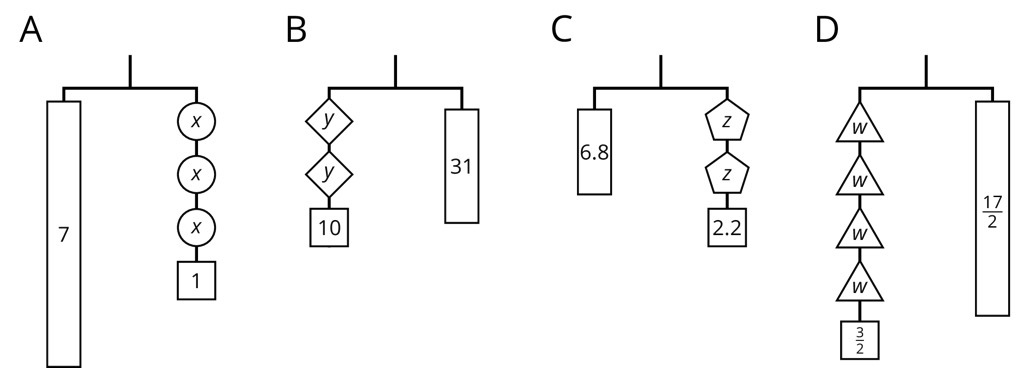PSABM6NF - Student Facing Task---Acc7.3 Lesson 7 Reasoning about Solving Equations (Part 1) (7.EE.B.4.a)
Part A)In the two diagrams, all the triangles weigh the same and all the squares weigh the same.

For each diagram, what is one thing that must be true.

Part B)

For each diagram, what is one thing that could be true.

Part C)

For each diagram, what is one thing that cannot possibly be true.

Part A)

On each balanced hanger, figures with the same letter have the same weight.Which hanger matches with the following equation:Select one:
Part B)

What letter belongs in the empty box of this equation:?

Select one:
Part C)

Which hanger matches with the following equation:Select one:
Part D)

What letter belongs in the empty box of this equation:?

Select one:
Part E)

Which hanger matches with the following equation:Select one:
Part F)

What letter belongs in the empty box of this equation:?

Select one:
Part G)

Which hanger matches with the following equation:Select one:
Part H)

What letter belongs in the empty box of this equation?

Select one:
Part I)

Solve for w in this equation.

w = ______

Type your answer below as a number (example: 5, 3.1, 4 1/2, or 3/2):
Part J)

Use the hanger to explain what the solution means.

Part K)

Solve for z in this equation.

z = ______

Type your answer below as a number (example: 5, 3.1, 4 1/2, or 3/2):
Part L)

Use the hanger to explain what the solution means.

Part M)

Solve for x in this equation.

x = ______

Type your answer below as a number (example: 5, 3.1, 4 1/2, or 3/2):
Part N)

Use the hanger to explain what the solution means.

Part O)

Solve for y in this equation.

y = ______

Type your answer below as a number (example: 5, 3.1, 4 1/2, or 3/2):
Part P)

Use the hanger to explain what the solution means.

Part A)Here are some balanced hangers where each piece is labeled with its weight.

Write an equation for Hanger A.

7 = ____________

Part B)

Write an equation for Hanger B.

____________ = 31

Part C)

Write an equation for Hanger C.

6.8 = ____________

Part D)

Write an equation for Hanger D.

____________ = 17/2